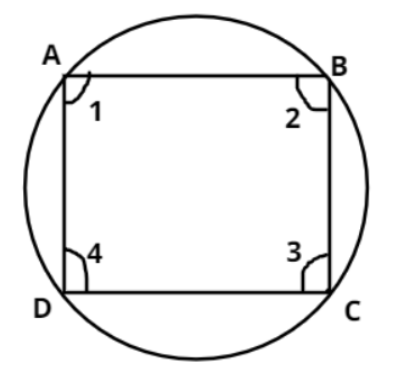Courses
Courses for Kids
Free study material
Free LIVE classes
MoreLIVE
Join Vedantu’s FREE Mastercalss

# Prove that a cyclic parallelogram is a rectangle.Verified
264.7k+ views
Hint: In order to prove that a cyclic parallelogram is a rectangle, we will prove one of the properties of a rectangle (i.e., all the interior angles of the rectangle are ${90^0}$) by using the concept of a cyclic parallelogram.Let us consider a cyclic parallelogram ABCD with interior angles of the parallelogram ABCD as $\angle 1,\angle 2,\angle 3{\text{ and }}\angle {\text{4}}$.
As we know that in any cyclic parallelogram, any two opposite angles are equal in measure and the sum of any two opposite pair of angles gives ${180^0}$.
i.e., $\angle 1 = \angle 3{\text{ }} \to {\text{(1)}}$ and $\angle 1 + \angle 3 = {180^0}{\text{ }} \to {\text{(2)}}$, $\angle 2 = \angle 4{\text{ }} \to {\text{(3)}}$ and $\angle 2 + \angle 4 = {180^0}{\text{ }} \to {\text{(4)}}$
Using equation (1) in equation (2), we have
$\angle 1 + \angle 1 = \angle 3 + \angle 3 = {180^0} \\ \Rightarrow 2\angle 1 = 2\angle 3 = {180^0} \\ \Rightarrow \angle 1 = \angle 3 = {90^0} \\$
Similarly, using equation (3) in equation (4), we have
$\angle 2 + \angle 2 = \angle 4 + \angle 4 = {180^0} \\ \Rightarrow 2\angle 2 = 2\angle 4 = {180^0} \\ \Rightarrow \angle 2 = \angle 4 = {90^0} \\$
Therefore, $\angle 1 = \angle 2 = \angle 3 = \angle 4 = {90^0}$ which means all the interior angles of the given cyclic parallelogram are ${90^0}$.
Also we know that all the interior angles of a rectangle are equal to ${90^0}$.
Hence, a cyclic parallelogram is a rectangle.

Note: In these types of problems, we will use the property of cyclic parallelogram that in any cyclic parallelogram, the sum of any two opposite pair of angles gives a measure of ${180^0}$ and any opposite pair of angles of the cyclic parallelogram are equal.
Last updated date: 01st Oct 2023
Total views: 264.7k
Views today: 6.64k